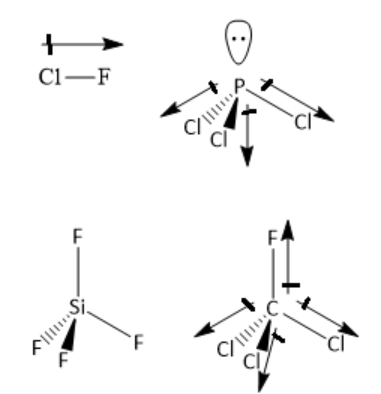QuestionAnswers

# Which of the following has zero dipole moment?A ) ${\text{ClF}}$B ) ${\text{PC}}{{\text{l}}_3}$C ) ${\text{Si}}{{\text{F}}_4}$D ) ${\text{CFC}}{{\text{l}}_3}$Verified
57k+ views
Hint: Based on the molecular geometry, determine if the individual bond dipoles cancels each other or not . If the individual bond dipoles cancels each other, the molecule is nonpolar, with zero dipole moment. If the individual bond dipoles do not cancel each other, the molecule is polar, with non-zero dipole moment.

${\text{ClF}}$ is a linear molecule as it has only two atoms. Two atoms are from two different elements having different electronegativities. Hence, the chlorine-fluorine bond is polar, and has a dipole moment. Hence, the molecule is a polar molecule and has a dipole moment.

In ${\text{PC}}{{\text{l}}_3}$ molecule, the central phosphorus atom has three bond pairs of electrons and one lone pair of electrons. It is $s{p^3}$ hybridised with trigonal pyramidal geometry. Phosphorus and chlorine atoms have different electronegativity. Hence, phosphorus chlorine bond is polar. The individual bond dipoles do not cancel each other. Hence, the molecule is polar with non zero dipole moment.

In ${\text{Si}}{{\text{F}}_{\text{4}}}$ the central silicon atom has four silicon-fluorine single bonds. The number of lone pairs of electrons is zero. The central silicon atom has four bonding domains and zero lone pairs of electrons. Hence, the silicon atom is $s{p^3}$ hybridised. The geometry around silicon atoms is tetrahedral. The individual bond dipoles cancel each other. Hence, it is a nonpolar molecule.

In ${\text{CFC}}{{\text{l}}_3}$ the central carbon atom has three carbon-chlorine single bonds and one carbon-fluorine single bond. The number of lone pairs of electrons is zero. The central carbon atom has four bonding domains and zero lone pairs of electrons. Hence, the carbon atom is $s{p^3}$ hybridised. The geometry around the carbon atom is tetrahedral. The electronegativity of carbon is different from that of fluorine or chlorine. Hence, carbon-chlorine and carbon-fluorine bonds are polar. The individual bond dipoles do not cancel each other. Hence, it is a polar molecule with non-zero dipole moment.

Thus, the molecule with zero dipole moment is silicon tetrafluoride ${\text{Si}}{{\text{F}}_4}$.Hence, the correct option is the option C ) ${\text{Si}}{{\text{F}}_4}$.

Note:
In heteronuclear diatomic / polyatomic molecules, polar bonds are present as atoms of different elements have different electronegativities. Not all molecules containing polar bonds have net dipole moment. There are some molecules containing polar bonds, yet these molecules have zero dipole moment. This is particularly true of symmetrical molecules in which individual bond dipoles cancel each other.